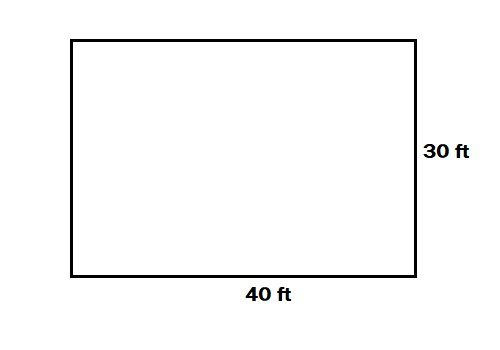# How To Calculate Quantity Of Soil/Sand For Filling Plot

How To Calculate Quantity Of Soil/Sand For Filling Plot:

Plot filling is one of the essential tasks in almost every construction project. So that the site gets developed and prepared for construction.

In this article, we will discuss how to calculate the quantity of soil or sand and the number of trally/tucks required for filling any plot or land.

Assuming,

• Length of the plot = 40 feet.
• Width of the plot = 30 feet.• Area of the plot = Length x Width = 40 x 30 =1200 sft.

Suppose we need to fill the plot by 3’5” = 3.41 feet.

So, the depth of the plot = 3.41 feet.

• Volume of plot to be filled = 1200 x 3.41 = 4092 cft.

This volume is for a loose state.

For full compacted state, required quantity of soil/sand for filling the plot = 4092 x 1.30 = 5319.6 cft= 5320 cft. (1.30 is factor)

Now we will calculate the required no of trally/ trucks.

Suppose a trally can carry 70 cft soil.

• ∴ No. of trally required = 5320/70 = 58.45 = 76 no. of trally.

Suppose a truck can carry 500 cft.

• ∴ No. of trucks required = 5320/500 = 11 no. of trucks.

Note: If your area has a complex shape you need to divide it into easy shapes then use the above method.

### 15 thoughts on “How To Calculate Quantity Of Soil/Sand For Filling Plot”

1. what if the land is not level, how can we get the volume of the site.

• Survey the land by autolevel find average of all the levels of plot

• Take average of leval

2. I like such civil engineering works because I am also a technical person working in the field and at construction site….

• if are Land like slope ( center are down to sides) so what will we estimate it’s clear.

3. Thanks to dailycivil for good work

4. Please define how to take the value of Factor of safe of soil compaction.

5. The factor 1.3 is from which code book.

6. Dailycivil is doing great job…
Thanks!

7. Does 1.3 a compaction factor in feet work also for metres?